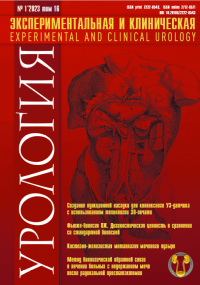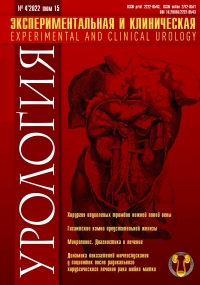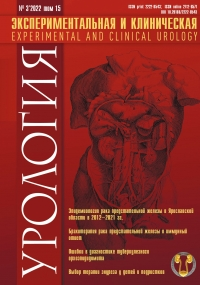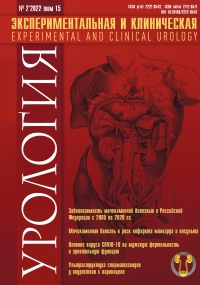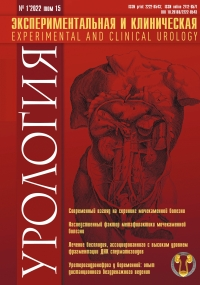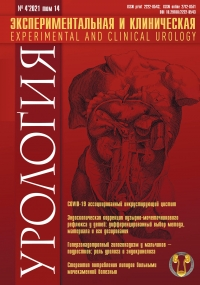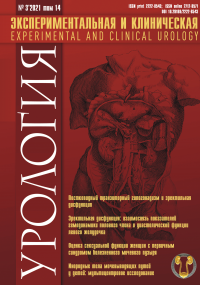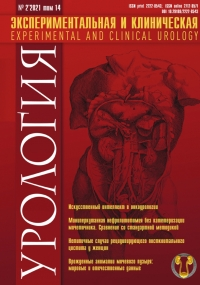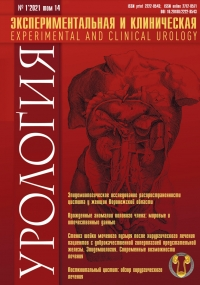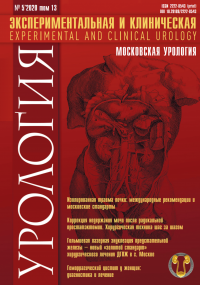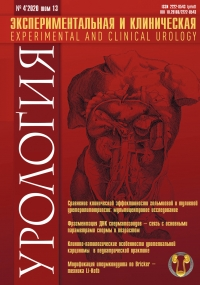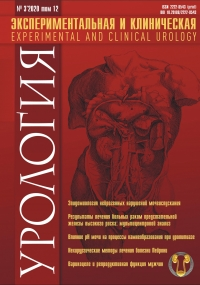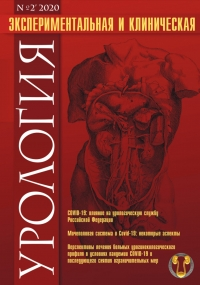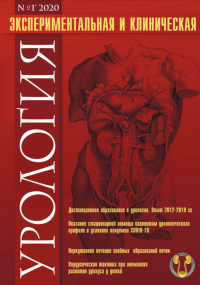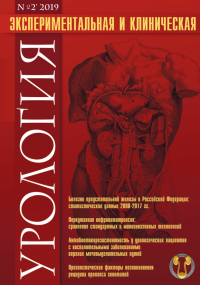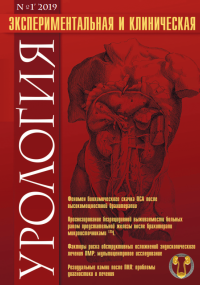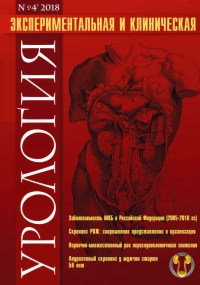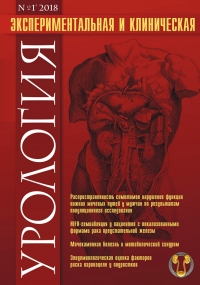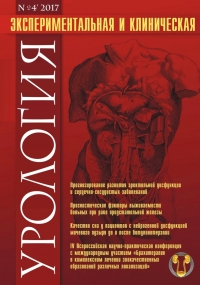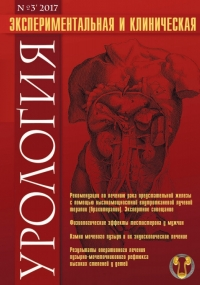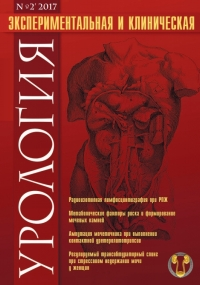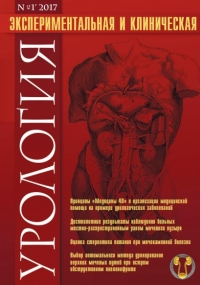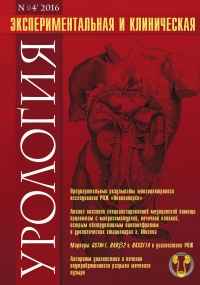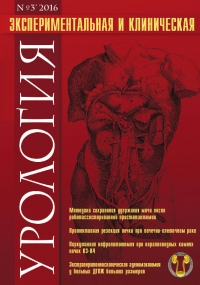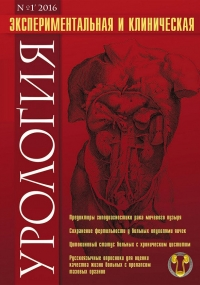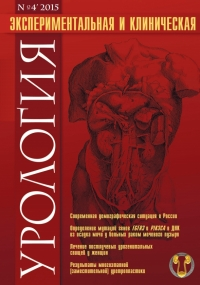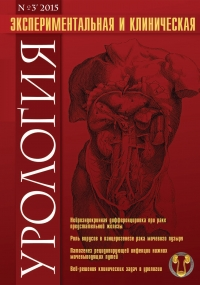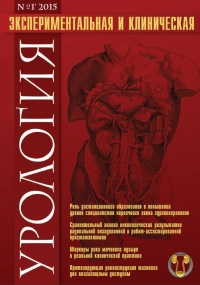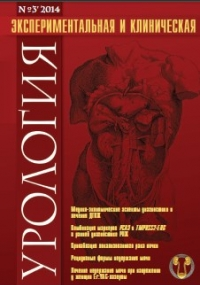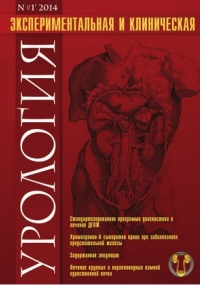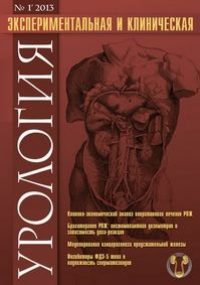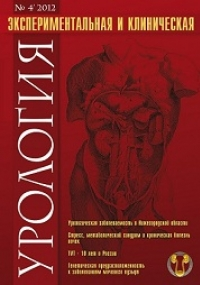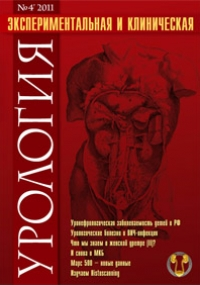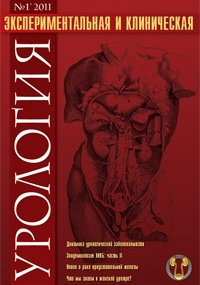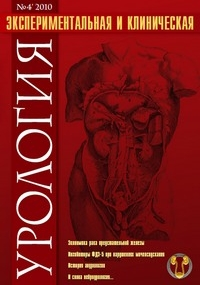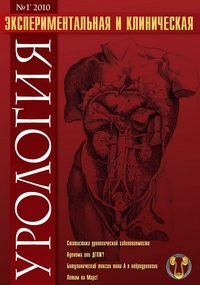Number №3, 2013 - page 79-82

# High-precision evaluation of systemic endothelial function in the complex diagnosis of erectile disorders

Efremov E.A., Dorofeev S.D., Mel'nik Ya.I., Kulikov A.O., Krasnyak S.S.3554

Introduction: It has been found that the leading pathophysiological mechanism of arteriogenic erectile dysfunction (ED) is a violation of the endothelial function of cavernous arteries. Given the central role of endothelial function in arteriogenic ED and often subjective indicators of endothelial function caused by, resulting from the postcompressive ultrasonic measurement technique requires a more precise and perfect. The evaluation of postcompressive reactive hyperemia index (RHI) is a modern diagnostic tool, which has a high precision determination of the state of endothelial function.

Materials and methods: We conducted an assessment of endothelial function in 126 men complaining of erectile dysfunction (42,6 ± 6,8 years).

Results: The regression analysis and determination of the Spearman rank correlation coefficient shown a high degree of correlation of endothelial dysfunction with increased blood glucose (r = 0,75), with a waist (r = 0,65), age (r = 0,63), increased triglycerides (r = 0,6), decreased high density cholesterol levels (r = 0,5), increased low density cholesterol (r = 0,48), reduced number of points by IIEF (r = 0,45), the lowest degree of association with the identified reduced peak systolic velocity (r = 0,3), low Index of resistance (r = 0,28), end diastolic velocity (r = 0,2), elevated blood pressure> 130 mm Hg (r = 0,2), retention time of blood flow in deep dorsal vein > 15 minutes (r = 0,1).

Conclusion: The evaluation of postcompressive reactive hyperemia index is a highly accurate method of assessing systemic endothelial function in the complex diagnosis of ED.

AttachmentSize
Скачать статью283.36 KB